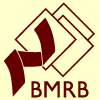# NMR Restraints Grid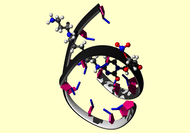Result table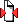(Save to zip file containing files for each block)

 image mrblock_id pdb_id bmrb_id cing stage position program type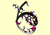571637 2mcj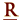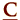19441 cing 1-original 4 unknown unknown

```!G1-C16
group
select= ((resid  1 and (name n1 or name c6 or name c2)) or
(resid 16 and (name n3)))
weight=10.0
end

group
select= ((resid  1 and (name n1)) or
(resid 16 and (name n3 or name c2 or name c4)))
weight=10.0
end

!T2-A15
group
select= ((resid 15 and (name n1 or name c6 or name c2)) or
(resid  2 and (name n3)))
weight=10.0
end

group
select= ((resid 15 and (name n1)) or
(resid  2 and (name n3 or name c2 or name c4)))
weight=10.0
end

!C3-G14
group
select= ((resid 14 and (name n1 or name c6 or name c2)) or
(resid  3 and (name n3)))
weight=10.0
end

group
select= ((resid 14 and (name n1)) or
(resid  3 and (name n3 or name c2 or name c4)))
weight=10.0
end

!G4-C13
group
select= ((resid  4 and (name n1 or name c6 or name c2)) or
(resid 13 and (name n3)))
weight=10.0
end

group
select= ((resid  4 and (name n1)) or
(resid 13 and (name n3 or name c2 or name c4)))
weight=10.0
end

!G5-C12
group
select= ((resid  5 and (name n1 or name c6 or name c2)) or
(resid 12 and (name n3)))
weight=10.0
end

group
select= ((resid  5 and (name n1)) or
(resid 12 and (name n3 or name c2 or name c4)))
weight=10.0
end

!C6-G11
group
select= ((resid 11 and (name n1 or name c6 or name c2)) or
(resid  6 and (name n3)))
weight=10.0
end

group
select= ((resid 11 and (name n1)) or
(resid  6 and (name n3 or name c2 or name c4)))
weight=10.0
end

!T7-A10
group
select= ((resid 10 and (name n1 or name c6 or name c2)) or
(resid  7 and (name n3)))
weight=10.0
end

group
select= ((resid 10 and (name n1)) or
(resid  7 and (name n3 or name c2 or name c4)))
weight=10.0
end

!G8-C9
group
select= ((resid  8 and (name n1 or name c6 or name c2)) or
(resid  9 and (name n3)))
weight=10.0
end

group
select= ((resid  8 and (name n1)) or
(resid  9 and (name n3 or name c2 or name c4)))
weight=10.0
end

end

```

Please acknowledge these references in publications where the data from this site have been utilized.

Contact the webmaster for help, if required. Thursday, October 21, 2021 2:25:56 AM GMT (wattos1)